# Electrical network

For electrical power transmission grids and distribution networks, see Electrical grid.A simple electric circuit made up of a voltage source and a resistor. Here,, according to Ohm's law.

An electrical network is an interconnection of electrical components (e.g. batteries, resistors, inductors, capacitors, switches) or a model of such an interconnection, consisting of electrical elements (e.g. voltage sources, current sources, resistances, inductances, capacitances). An electrical circuit is a network consisting of a closed loop, giving a return path for the current. Linear electrical networks, a special type consisting only of sources (voltage or current), linear lumped elements (resistors, capacitors, inductors), and linear distributed elements (transmission lines), have the property that signals are linearly superimposable. They are thus more easily analyzed, using powerful frequency domain methods such as Laplace transforms, to determine DC response, AC response, and transient response.

A resistive circuit is a circuit containing only resistors and ideal current and voltage sources. Analysis of resistive circuits is less complicated than analysis of circuits containing capacitors and inductors. If the sources are constant (DC) sources, the result is a DC circuit.

A network that contains active electronic components is known as an electronic circuit. Such networks are generally nonlinear and require more complex design and analysis tools.

## Classification

### By passivity

An active network is a network that contains an active source - either a voltage source or current source.

A passive network is a network that does not contain an active source.

### By linearity

A network is linear if its signals obey the principle of superposition; otherwise it is non-linear.

## Classification of sources

Sources can be classified as independent sources and dependent sources

### Independent

Ideal Independent Source maintains same voltage or current regardless of the other elements present in the circuit. Its value is either constant (DC) or sinusoidal (AC). The strength of voltage or current is not changed by any variation in connected network.

### Dependent

Dependent Sources depend upon a particular element of the circuit for delivering the power or voltage or current depending upon the type of source it is.

## Electrical laws

A number of electrical laws apply to all electrical networks. These include:

• Kirchhoff's current law: The sum of all currents entering a node is equal to the sum of all currents leaving the node.
• Kirchhoff's voltage law: The directed sum of the electrical potential differences around a loop must be zero.
• Ohm's law: The voltage across a resistor is equal to the product of the resistance and the current flowing through it.
• Norton's theorem: Any network of voltage or current sources and resistors is electrically equivalent to an ideal current source in parallel with a single resistor.
• Thévenin's theorem: Any network of voltage or current sources and resistors is electrically equivalent to a single voltage source in series with a single resistor.
• superposition theorem: In a linear network with several independent sources, the response in a particular branch when all the sources are acting simultaneously is equal to the linear sum of individual responses calculated by taking one independent source at a time.

Other more complex laws may be needed if the network contains nonlinear or reactive components. Non-linear self-regenerative heterodyning systems can be approximated. Applying these laws results in a set of simultaneous equations that can be solved either algebraically or numerically.

## Design methods

Linear network analysis
Elements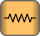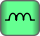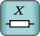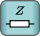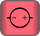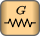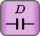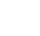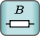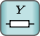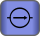Components
Series and parallel circuits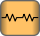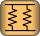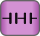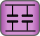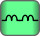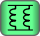Impedance transforms
Generator theorems Network theorems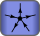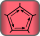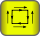Network analysis methods
Two-port parameters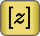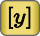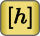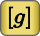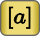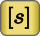To design any electrical circuit, either analog or digital, electrical engineers need to be able to predict the voltages and currents at all places within the circuit. Simple linear circuits can be analyzed by hand using complex number theory. In more complex cases the circuit may be analyzed with specialized computer programs or estimation techniques such as the piecewise-linear model.

Circuit simulation software, such as HSPICE (an analog circuit simulator), and languages such as VHDL-AMS and verilog-AMS allow engineers to design circuits without the time, cost and risk of error involved in building circuit prototypes.

## Network simulation software

More complex circuits can be analyzed numerically with software such as SPICE or GNUCAP, or symbolically using software such as SapWin.

### Linearization around operating point

When faced with a new circuit, the software first tries to find a steady state solution, that is, one where all nodes conform to Kirchhoff's current law and the voltages across and through each element of the circuit conform to the voltage/current equations governing that element.

Once the steady state solution is found, the operating points of each element in the circuit are known. For a small signal analysis, every non-linear element can be linearized around its operation point to obtain the small-signal estimate of the voltages and currents. This is an application of Ohm's Law. The resulting linear circuit matrix can be solved with Gaussian elimination.

### Piecewise-linear approximation

Software such as the PLECS interface to Simulink uses piecewise-linear approximation of the equations governing the elements of a circuit. The circuit is treated as a completely linear network of ideal diodes. Every time a diode switches from on to off or vice versa, the configuration of the linear network changes. Adding more detail to the approximation of equations increases the accuracy of the simulation, but also increases its running time.Wikimedia Commons has media related to electric circuits.Look up electrical circuit in Wiktionary, the free dictionary.